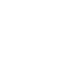# Apr 4 ACT & SAT Question of the Day

If you are reading this in an email you received from me, do not click the link to sat.collegeboard.org below. Use the link to my website that is farther down on the email. If you are seeing this in my blog, do the SAT Question of the Day by clicking on this link:

http://sat.collegeboard.org/practice/sat-question-of-the-day?src=R&questionId=20130404 (This link takes you to today’s question. If you use my archive, you will see the question related to my SAT explanation for that date.)

The answer is B.  If you’ve read the SAT staff’s explanation, I bet your head is still spinning!  You can certainly do it that way but why waste the time?  There’s got to be an easier way.  Yes there is.  Using the Wizard’s strategy of “changing the abstract to the concrete” makes this a simple question.  Set AB=1, BC=2, CD=3, and DE=4.  Now plug in the real numbers into the answers.  Answer A, for example, becomes 3<3 and that’s not true, so eliminate it.  Answer B becomes 3<7; that works.  Answer C becomes 6<7; that works.  Answer D becomes 6<4; that doesn’t work.  Answer E becomes 5<4; that doesn’t work.  We are left with B and C.  How do we decide?

There’s a few ways to decide which one MUST be true.  For Answer B, AB has to be smaller than CD and BC has to be smaller than DE; so, that is going to be the right answer but how do we eliminate Answer C? What if you substitute different numbers: AB=5, BC=6, CD=7, and DE=8?   Now Answer C doesn’t work.

My strategy often eliminates all the wrong answers on the first try.  On the other hand, as with this question, sometimes it doesn’t and you’re left with two answers.  Then you need to substitute different numbers and try again.  My new numbers worked to eliminate C.  You could have picked 2, 3, 4, and 5 and those values would have also eliminated Answer C.

Here’s something else you could have done and would have probably tried if you practice this strategy.  Don’t always use integers.  You could have made the line segments 1, 2, 2.1 and 2.2.  Those values would meet the rule we are given: AB<BC<CD<DE.  They don’t work for Answer C.  They must work for Answer B.  We are all done.

I’m hoping you find my tips, tricks, and insider secrets helpful when you can’t do the math or when doing the math takes too long.  With practice, you’ll often find them much more efficient ways to attack the questions.  Practicing is going to raise your confidence and, in turn, confidence will raise your score.  That is why I encourage you to practice a little bit every day.

Let’s move on to the ACT question.

http://www.act.org/qotd/ (The ACT staff does not put a date on their questions so if you click on an archived blog, you’ll get today’s question and the old explanation. Sorry. The SAT staff has dated their questions; so, the archive is helpful. The ACT folks simply don’t do that.)

The answer is C.  I hope the ACT explanation made sense to you because it sure doesn’t make sense to me!  I can see what they did but they needed to provide more details so that it truly is comprehensible.  Showing the missing step of canceling 24 minutes in the denominator with 60 minutes in the numerator so that you have: 4.8 mi times 2.5/1 hour helps see what they did.

I did it a very different way.  Always set up your calculations using the units that you are given in the question so that they relate to the units in the answer.  In this question, you need Distance/Time.  However, you start with miles/minute and that needs to end up as miles per hour.  So, begin by changing 24 minutes to hours by dividing 24 minutes/60 minutes = .4 hours.  Then 4.8 miles/.4 hours = 12 miles per hour, Answer C.  That’s probably the easiest way if you use your calculator.

I did it in my head without a calculator.  Remember that the test writers use numbers that are easy to manipulate.  They picked 24 for a reason; 12 minutes is 1/5 of an hour and 24 is therefore 2/5 of an hour.  (You can easily memorize 5 minutes is 1/12, 10 is 1/6, 15 is 1/4, and 20 is 1/3.)  That means that if you go 4.8 miles in 2/5 of an hour, you go 2.4 in 1/5.  There are 5 fifths in an hour so 5 times 2.4 is 12.  All done.

Keep practicing and you’ll see the results: higher scores!  You can’t cram for the tests; so, you need to do a little work each day.  Focus on the things that keep coming up on the test.  Doing these Questions of the Day helps.  Also get some real practice tests.  The SAT and ACT folks give free ones to your counselor.  Get them and use them.  To prepare really well, buy the Official SAT Study Guide and the Real ACT Prep Guide.  They are the only sources of actual tests with true scoring charts.  Send me an email if you see any questions that stump you.  I’ll be happy to help.

The Wizard